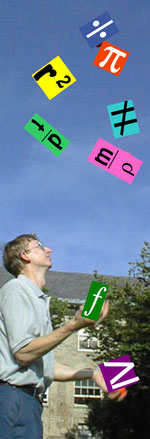# How do I isolate x (or P or T...) in a formula?Rearranging equations to solve for a given variable

## Equations as important geological tools

Sometimes, it may seem like your geoscience instructor is speaking another language when he/she talks about equations or formulae. Especially if he/she expects you to "manipulate" or rearrange them! But, equations can provide powerful tools for describing the natural world. In the geosciences, we can describe the behavior of many natural phenomena by writing an equation for a line (y = mx + b), or with exponential functions (y = ext). And with a little algebra, we can rearrange those equations to solve for ANY of the variables in them.

Although this may seem like magic, you don't have to be a "mathemagician" to do this. This page is designed to give you some tools to call upon to help you to learn some simple steps to help you to solve an equation for any of the variables (letters that represent the element or quantity of interest).

## Why should I manipulate equations?Believe it or not, there are many good reasons to develop your ability to rearrange equations that are important to the geosciences. It can save time, help you with units and save some brain space! Here are some reasons to develop your equation manipulation skills (in no particular order):

• Equations are easier to handle before inserting numbers! And, if you can isolate a variable on one side of the equation, it is applicable to every similar problem that asks you to solve for that variable!
• If you know how to manipulate equations, you only have to remember one equation that has all the variables of question in it - you can manipulate it to solve for any other variable! This means less memorization!
• Manipulating equations can help you keep track of (or figure out) units on a number. Because units are defined by the equations, if you manipulate, plug in numbers and cancel units, you'll end up with exactly the right units (for a given variable)!

## Where is this used in the geosciences?

To be honest, equation manipulation occurs in almost every aspect of the geosciences. Any time you see a P or T or ρ or x (or even =), there is an equation that you could manipulate. Because equations can be used to describe lots of important natural phenomena, being able to manipulate them gives you a powerful tool for understanding the world around you!

See the Practice Manipulating Equations page for just a few examples.

## A Review of Important Rules for Rearranging Equations

You probably learned a number of rules for manipulating equations in a previous algebra course. It never hurts to remind ourselves of the rules. So let's review:

## Some simple steps for manipulating equationsHere are some simple steps for manipulating equations. Under each step you will find an example of how to do this with an example that uses the geologic context of density (a measure of mass per unit volume).

1. Assess what you have (which of the variables do you have values for?, what units are present?, etc.). DO NOT plug in any numbers yet!
2. Determine which of the variables you want as your answer. (What is the question asking you to calculate? What is the unknown variable?)
3. Rearrange the equation so that the unknown variable is by itself on one side of the equals sign (=) and all the other variables are on the other side. RULE #1: you can add, subtract, multiply and divide by anything, as long as you do the same thing to both sides of the equals sign.
4. NOW plug in the numbers! Replace known variables with their values and don't forget to keep track of units!
5. Determine the value of the unknown variable by performing the mathematical functions. That is, add, subtract, multiply and divide according to the equation you wrote for step 2.
6. Ask yourself whether the answer is reasonable in the context of what you know about the geosciences and how much things should weigh.

## More help with equations

The chemistry department at Texas A&M has a math review page about Algebraic Manipulation

The Economics and Business faculty at University of Sidney has a page where you can practice your equation manipulation skills! Take the algebraic manipulation quizzes!

This page was written and compiled by Dr. Jennifer M. Wenner, Geology Department, University of Wisconsin Oshkosh and Dr. Eric M. Baer, Geology Program, Highline Community College